Next: Inexact top K document Up: Computing scores in a Previous: Computing scores in a   Contents   Index

# Efficient scoring and ranking

We begin by recapping the algorithm of Figure 6.14 . For a query such as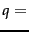jealous gossip, two observations are immediate:

1. The unit vector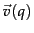has only two non-zero components.
2. In the absence of any weighting for query terms, these non-zero components are equal - in this case, both equal 0.707.

For the purpose of ranking the documents matching this query, we are really interested in the relative (rather than absolute) scores of the documents in the collection. To this end, it suffices to compute the cosine similarity from each document unit vector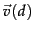to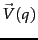(in which all non-zero components of the query vector are set to 1), rather than to the unit vector. For any two documents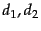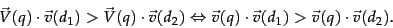(34)

For any document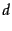, the cosine similarity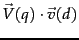is the weighted sum, over all terms in the query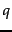, of the weights of those terms in. This in turn can be computed by a postings intersection exactly as in the algorithm of Figure 6.14 , with line 8 altered since we take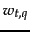to be 1 so that the multiply-add in that step becomes just an addition; the result is shown in Figure 7.1 . We walk through the postings in the inverted index for the terms in, accumulating the total score for each document - very much as in processing a Boolean query, except we assign a positive score to each document that appears in any of the postings being traversed. As mentioned in Section 6.3.3 we maintain an idf value for each dictionary term and a tf value for each postings entry. This scheme computes a score for every document in the postings of any of the query terms; the total number of such documents may be considerably smaller than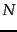.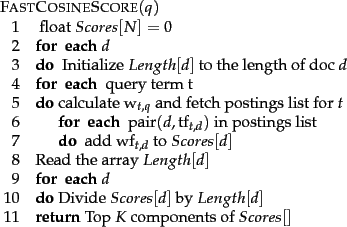Given these scores, the final step before presenting results to a user is to pick out the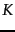highest-scoring documents. While one could sort the complete set of scores, a better approach is to use a heap to retrieve only the topdocuments in order. Where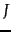is the number of documents with non-zero cosine scores, constructing such a heap can be performed in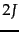comparison steps, following which each of thehighest scoring documents can be read off'' the heap with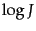comparison steps.

SubsectionsNext: Inexact top K document Up: Computing scores in a Previous: Computing scores in a   Contents   Index